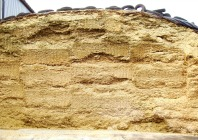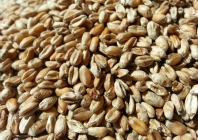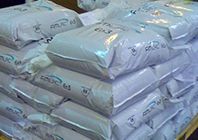# Knowing which feed to use

Formulating the FiMLAC way makes feed choice quite simple (once you know how!).  In the traditionally formulated ration example (ExampleRationHighPtn PDF), the 22% compound was formulated to the crude protein content. It happened to contain 108g/kg MPE and 151g/kg MPN.

### MPE

In the ration report (ExampleRationHighPtn PDF), the Milk Yield Potential from MP is 3 litres short of the ME supply.  It is roughly 46g of MP needed for each litre of milk, 3 x 46 = 138g short.  Divided by the 12kg of compound being fed = 11.5g/kg short of MPE.  Our compound contains 108.0g/kg + 11.5g/kg extra  = 119.5g/kg needed in the feed.

Compare this to the well balanced ration (ExampleRationBalanced PDF) where the FiMLAC 120M has MPE at 120g/kg as standard.

### MPN

In the example ration (ExampleRationHighPtn PDF), the excess MPN is far too high at 468g/day.  Ideally, we would be below 300g/day, so a need to lose 168g/day out of the ration, i.e., lose 168g in 12kg of compound = 14g/kg less excess MPN in the compound.

The 22% compound as formulated has 151g/kg MPN and 108g/kg MPE, so an excess MPN of 43g/kg.  So, we now need a maximum MPN of 43 – 14 = 29g/kg above the new MPE of 120g/kg, so 149g/kg MPN.

Compare FiMLAC 120M which has the MPN set at 145g/kg as standard.

In a way, it is just like comparing tractor engine sizes!

Using the FiMLAC approach, the guesswork is taken out of the equation.FiMLAC RangeForage AnalysisGrain ExchangeOur Shops